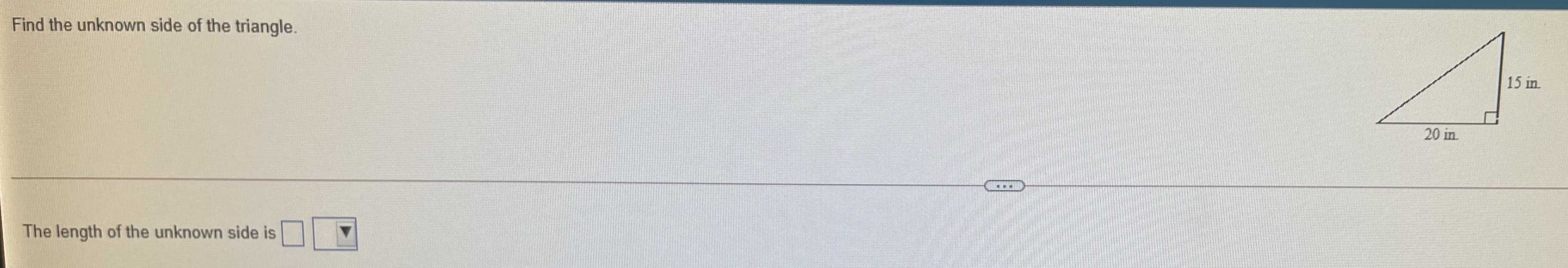### ¿Todavía tienes preguntas de matemáticas?

Pregunte a nuestros tutores expertos
Trigonometry
PreguntaFind the unknown side of the triangle. The length of the unknown side is $$\square$$

$$= \sqrt{20^{2} + 15^{2} } = 25$$